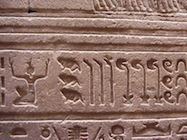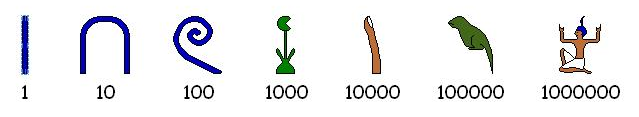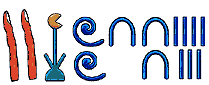# Numeric EncodingHow can numbers be stored, and operated upon, by machines?

## Numbers and Numerals

A number is an abstraction of quantity. Humans started with counting numbers like one, two, three, four and so on. Zero came later. Then someone realized that subtracting six from two could be useful and so invented negative numbers. Then integer division led to the creation of rational numbers, and other work resulted in irrational numbers, real numbers, transfinite numbers, imaginary numbers, complex numbers and so on.

A number is represented by a numeral.

## Egyptian Numerals

Egyptians wrote numerals in hieroglyphs for thousands of years; here are the important symbols and their numeric values:Notice that these numerals could be written from right-to-left or left-to-right or vertically or a combination of vertical and horizontal. Here is one way to represent twenty-one thousand two hundred thirty-seven:We don’t use these numerals in computer systems today, but history is important, so please read more about these numerals at Wikipedia or elsewhere.

Exercise: Write out the number 5,994,998,999 using heiroglyphics. (Just kidding.)

## Roman Numerals

Well, these aren’t much use in modern computers, either. Enough said. Besides, they’re really hard to understand, and they don’t have a zero, or wait...maybe they do?

## Positional Numeral Systems

A Positional Numeral System has an ordered set of digits that includes 0 as its first member. The number of digits in the set is called the base. By far the most common of these is the Hindu-Arabic Numeral System, or Arabic Numeral System, which has a base of 10, and whose glyphs are these:

  0  1  2  3  4  5  6  7  8  9


There are other positional systems, too:

• Devanagari (base 10): ० १ २ ३ ४ ५ ६ ७ ८ ९
• Mongolian (base 10): ᠐ ᠑ ᠒ ᠓ ᠔ ᠕ ᠖ ᠗ ᠘ ᠙
• Kannada (base 10): ೦ ೧ ೨ ೩ ೪ ೫ ೬ ೭ ೮ ೯
• Tamil (base 10): ௦ ௧ ௨ ௩ ௪ ௫ ௬ ௭ ௮ ௯
• Maya (base 20)
• Kaktovik Iñupiaq (base 20)

In computing, though, the Hindu-Arabic numerals dominate. We can extend this system to bases other than 10 by adding or removing glyphs. Bases up to 36 are traditionally handled by adding the Latin letters A-Z. The most common systems in computing are:

• Decimal: digit set = {0,1,2,3,4,5,6,7,8,9}, base = 10.
• Octal: digit set = {0,1,2,3,4,5,6,7}, base = 8.
• Hexadecimal: digit set = {0,1,2,3,4,5,6,7,8,9,A,B,C,D,E,F}, base = 16.
• Binary: digit set = {0,1}, base = 2.

You generate positional numerals starting at 0 by a really easy algorithm you already know.

DecimalOctalHexBinary
0 0 0 0
1 1 1 1
2 2 2 10
3 3 3 11
4 4 4 100
5 5 5 101
6 6 6 110
7 7 7 111
8 108 1000
9 119 1001
1012A 1010
1113B 1011
1214C 1100
1315D 1101
1416E 1110
1517F 1111
16201010000
17211110001
18221210010
19231310011
20241410100
21251510101
22261610110
23271710111
24301811000
25311911001
26321A11010
27331B11011
28341C11100
29351D11101
30361E11110
31371F11111
324020100000
334121100001
............
Third Graders, Binary Numbers, and the Socratic Method

### Conversion

Let’s convert Hex to and from Binary. Wait, this is trivial. Each group of four bits maps exactly to one hex digit. MEMORIZE THIS MAPPING SO YOU CAN DO THE CONVERSION BY SIGHT. Examples:

48C = 0100 1000 1100
CAFE54 = 1100 1010 1111 1110 0101 0100


Hex to Decimal: All Arabic numeral systems use place values which you should already be aware of. Examples:

E2A = 14(162) + 2(161) + 10(160)
= 14(256) + 2(16) + 10
= 3626


For numbers involving only two hex-digits you can do this in your head if you have memorized your multiples of 16: 0→0, 1→16, 2→32, 3→48, 4→64, 5→80, 6→96, 7→112, ... F→240. This gives you the contribution of the first hex digit, so just “add in” the second.

Decimal to Hex: Keep on dividing by 16, working your way backwards. Example:

       3653
/    \
228     5
/   \
14    4
/  \
0   14

==> E45


Binary to Decimal: Read left-to-right, doubling as you go and adding one where necessary. Example:

10110110


Say “1, 2, 5, 11, 22, 45, 91, 182.” Very easy! Of course, once you’re a pro you might just say “176 + 6 = 182” because you immediately see the 8 bits as a 1011 and a 0110, i.e., an 11 and a 6, and 16×11 is 176, etc.

This method is, in some circles, known as Dorin’s Way.

What about conversion between arbitrary bases? Well, it’s pretty cool to know how to generic conversions, but our focus today is stricly on binary, hex, and decimal.

You learned the addition method a long time ago for decimal numerals; the same idea applies to other bases.

#### Examples in Binary

 11010        101111       001        01
01101        111101       111        00
100111       1101100      1000        01


#### Examples in Hex

D371        9        37EE        89CA
26A2        9          F0        CC18
FA13       12        38DE       155E2


### Subtraction, Multiplication, and Division

You probably learned algorithms for these operations, long ago; the good news is that they are base-independent. Try some of these out in binary: you'll find they're quite simple, though they might take longer since there are so many bits, even for small quantities.

## Encoding Integers in Fixed Length Bit Strings

Computers have storage locations with a fixed number of bits. The bits are numbered starting at the right. Examples:

 3 2 1 0 1 1 0 0
 7 6 5 4 3 2 1 0 1 0 1 0 0 1 1 1
 15 14 13 12 11 10 9 8 7 6 5 4 3 2 1 0 0 0 1 1 1 1 1 1 0 0 1 0 0 1 0 1

Storage locations come in many sizes. Usually we write the values in a storage location out in hex; the contents of our above examples are C, A7, and 3F25, respectively.

An $n$-bit storage location can store $2^n$ distinct bit strings so it can encode (“unsigned”) integers from 0 through $2^n-1$. If we want to include some negative numbers (“signed”) we have several encoding options, but by far the most common is called the Two’s complement representation, which allocates the bit strings to the numbers $-2^{n-1}$ through $2^{n-1}-1$. Please note that a given bit string can be interpreted as either an unsigned number or a signed one.

Here’s how things look in a four-bit storage location, with signed values using the two’s complement representation:

BinaryHex Unsigned
Decimal
Signed
Decimal
00000 0 0
00011 1 1
00102 2 2
00113 3 3
01004 4 4
01015 5 5
01106 6 6
01117 7 7
10008 8-8
10019 9-7
1010A10-6
1011B11-5
1100C12-4
1101D13-3
1110E14-2
1111F15-1

For 32-bit locations there are 4294967296 possible bit strings; here are some of them:

BinaryHex Unsigned
Decimal
Signed
Decimal
000000000000000000000000000000000000000000
000000000000000000000000000000010000000111
............
0110100010101111111000010000110168AFE10D17563568771756356877
............
011111111111111111111111111111107FFFFFFE21474836462147483646
011111111111111111111111111111117FFFFFFF21474836472147483647
10000000000000000000000000000000800000002147483648-2147483648
10000000000000000000000000000001800000012147483649-2147483647
............
1001011101010000000111101111001197501EF32538610419-1756356877
............
11111111111111111111111111111110FFFFFFFE4294967294-2
11111111111111111111111111111111FFFFFFFF4294967295-1

Here are the values that can represented in locations of different sizes:

BitsUnsigned RangeSigned Range
800…FF
0…255
80…7F
-128 …127
160000…FFFF
0 …65,535
8000…7FFF
-32,768 …32,767
24000000…FFFFFF
0…16,777,215
800000…7FFFFF
-8,388,608…8,388,607
3200000000…FFFFFFFF
0…4,294,967,295
80000000…7FFFFFFF
-2,147,483,648…2,147,483,647
640000000000000000…FFFFFFFFFFFFFFFF
0…18,446,744,073,709,551,615
8000000000000000…7FFFFFFFFFFFFFFF
-9,223,372,036,854,775,808
…9,223,372,036,854,775,807

With adding numbers using fixed length storage locations, our sum might not fit. This is called overflow.

Example: 8-bit location, unsigned numbers. Add C5 + EE. The value should be 1B3, but that won’t fit! Why? (Note: in decimal, this is adding 197 + 238 = 435, which is outside the range of 0..255.)

There are two main approaches to dealing the problem of a sum not being able to fit. We could

• Clamp the sum at the maximum representable value. This is called saturated arithmetic. In the previous example the sum would be FF (or 255 decimal).
• Ignore the carry, making our example’s result as B3. This is called modular (or wraparound) arithmetic.

What about signed numbers? Example: Try 6C + 53. You get 6C + 53 = BF. Well, you didn’t carry anything out when you added, and the answer still fits in 8 bits, but you added two positive numbers and got a negative result. This too, is overflow. Again we could

• Clamp the sum at the maximum representable value (which in the above example is 7F).
• Leave the result as BF.

Almost all computers do modular arithmetic. Some do modular and saturated arithmetic (e.g. In the Intel x86 family modular addition is done with the add or padd instructions, and saturated addition is done with padds or paddus instructions.

#### Overflow Detection

You have to know how to detect overflow. For unsigned numbers this occurs precisely when you carry out of the highest order bit. For signed numbers this occurs precisely when you’ve added two positive numbers and got a negative result, or added two negative numbers and got a positive result.

Examples of signed modular addition in 8-bit storage locations

    2C       78       42       E0       87       FF
38       6A       FC       75       DA       C1
64       E2       3E       55       61       C0

cry:no   cry:no   cry:yes  cry:yes  cry:yes  cry:yes
ovf:no   ovf:yes  ovf:no   ovf:no   ovf:yes  ovf:no


### Subtraction for fixed-length Integers

Because of the cool way the two’s complement representation works, you can subtract a-b by computing a+(-b). So just find the additive inverse of b and add it to a. By the way someone with a really sick sense of humor called the additive inverse the two’s complement and the name stuck. Finding the additive inverse is easy: just invert every bit and add 1!

Hold on! Before you start doing this, MAKE SURE YOU UNDERSTAND WHY THIS TECHNIQUE IS CORRECT!

Example in 8 bits: 44 decimal is 2C in hex or 00101100 in binary. So -44 decimal is found like this

00101100   =====invert bits=====>   11010011
1
11010100   ==> D4.


So this says that 2C and D4 are inverses. To do a sanity check, add them together and make sure you get zero. Check: 2C+D4=00.

A subtraction example in 8 bits:

E2-83 = E2+(-83) = E2+7D = 5F


By the way, if you start “thinking in hex”, you can do this stuff much faster. We’ll see better techniques in class.

### Prefix Multipliers for Sizes in Bytes

When numbers get really large you can use some special values:

TensTwos
kkilo103 Thousand Kikibi2101,024
Mmega106 MillionMimebi2201,048,576
Ggiga109 BillionGigibi2301,073,741,824
Ttera1012Trillion Titebi2401,099,511,627,776
Eexa 1018QuintillionEiexbi2601,152,921,504,606,846,976
Zzetta 1021Sextillion Zizebi2701,180,591,620,717,411,303,424
Yyotta 1024Septillion Yiyobi2801,208,925,819,614,629,174,706,176
Xxona 1027Octillion Xixobi2901,237,940,039,285,380,274,899,124,224
Wweka 1030Nonillion Wiwebi21001,267,650,600,228,229,401,496,703,205,376
Vvunda 1033Decillion Vivubi21101,298,074,214,633,706,907,132,624,082,305,024
Uuda 1036UndecillionUiudbi21201,329,227,995,784,915,872,903,807,060,280,344,576

(Everything up to yotta is part of the official SI system of units. The xona, weka, etc. prefixes are being looked at; alternatives for $10^{27}$ and $10^{30}$ are nona and dogga ... because it’s fun to say “doggabyte.”) Possibly even better: There is an online petition you can sign to get the SI to make “hella” the official prefix for 1027.

Examples
  4 Ki   = 22210 = 212 = 4096
64 Ki  = 26210 = 216 = 65536
256 Ki = 28210 = 218 = 262144
16 Mi  = 24220 = 224 = 16777216
4 Gi   = 22230 = 232 = 4294967296
32 Ti  = 25240 = 245 = 35184372088832
2 Pi   = 21250 = 251 = 2251799813685248

1 EiB is 1024 PiB.

Exercise: Find out how much printed information exists in the world; express the value in exabytes.
Exercise: How many exabytes worth of information has Facebook amassed?

## Real Numbers

A real number is, well, I leave it to you to look up its formal mathematical definition because it isn’t really trivial. But you should have some idea. The important thing here is: how can we represent them in fixed-length storage locations? We could use integer pairs for numbers we know to be rational, or take a long string and assume the decimal point is always in a certain place (called a fixed-point representation), or use a floating-point representation.

## Encoding Floating Point Numbers in Fixed Length Bit Strings

Most modern general purpose computers use an encoding scheme for floating point values called IEEE 754. There are (at least) two representations. One uses 32-bits and the other uses 64-bits.

### IEEE 754 Single-Precision (32 bits) Representation

The thirty-two bits are broken into three sections, the sign (s), the fraction (f), and the exponent (e).

 31 30 23 22 0 s e f

Taking s, f, and e as unsigned values, the value of the number, in decimal, is

efsvalue
1..254anything0(1 + f × 2-23) × 2e-127
1-(1 + f × 2-23) × 2e-127
000+0
1-0
not 00(f × 2-23) × 2-126
1-(f × 2-23) × 2-126
25500+infinity
1-infinity
1...222-1anythingSignaling NaN
222...223-1Quiet NaN

Example: What does C28A8000 represent? In binary, it is

    1 10000101 00010101000000000000000


So, s = 1, e = 133. Our value is then -(1.00010101)2 × 26 = -(1000101.01)2 which is clearly -69.25 in decimal.

### IEEE 754 Double-Precision (64 bits) Representation

The sixty-four bits are broken into three sections, the sign (s), the fraction (f), and the exponent (e).

 63 62 52 51 0 s e f

Taking s, f, and e as unsigned values, the value of the number, in decimal, is

efsvalue
1..2046anything0(1 + f × 2-52) × 2e-1023
1-(1 + f × 2-52) × 2e-1023
000+0
1-0
not 00(f × 2-52) × 2-1022
1-(f × 2-52) × 2-1022
204700+infinity
1-infinity
1...251-1anythingSignaling NaN
251...252-1Quiet NaN

### Special Floating Point Values

NaN means “not a number.” Quiet NaNs (QNaNs) propagate happily through computations. Signaling NaNs (SNaNs) can be used to signal serious problems. You can use them to indicate uninitialized variables, for example.

• Overflowing addition, subtraction, multiplication gives the expected infinity
• Adding two positive infinities gives positive infinity
• Adding two negative infinities gives negative infinity
• But infinity minus infinity = NaN
• A finite value divided by an infinity = 0
• Any nonzero value divided by 0 = infinity
• But 0 / 0 = NaN
• infinity × 0 = NaN
• infinity × infinity = infinity
• infinity / infinity = NaN
• Any arithmetic operation on a NaN results in NaN
• A NaN never compares equal to anything, not even another NaN